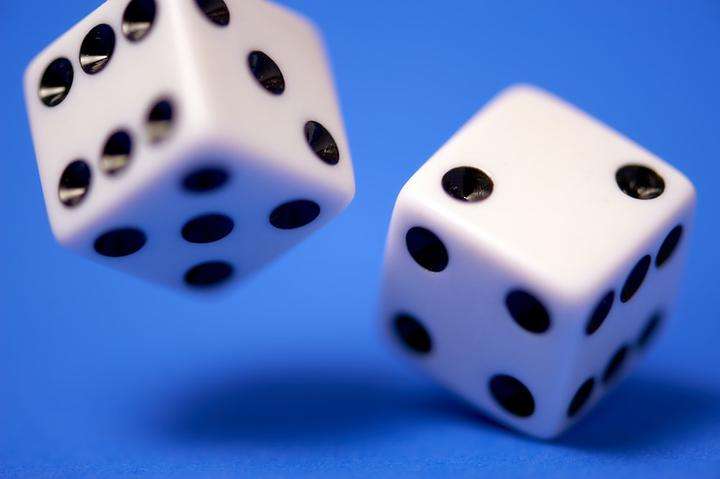# Introduction to ProbabilityProbability is at the heart of statistics, as most of the time, we won’t have the entire dataset to work on. Still, instead, we will need to understand how the population works relying on a sample. From these samples, we can calculate mean, median, standard deviations etc., from the observed frequencies, as they are estimates of unknown quantities that we haven’t observed.

With reasonable assumptions and without directly observe the property of interest, we can create a theoretical model that reproduces the observed frequency distribution of interest. We call these models probabilistic models.

Every experiment has a probabilistic model when we establish:

• Sample space $$\Omega$$
• Set of all possible outcomes for a given experiment
• Probability of $$\mathbb{P}(\omega)$$ for each element of $$\Omega$$, i.e., we want the probability of a random event or event

Example: We throw a fair coin twice. If H indicates heads and T indicates tails, the sample space is:

$\Omega = \{\omega_1, \omega_2, \omega_3, \omega_4\},$

Where $$\omega_1 = (H, H)$$, $$\omega_2 = (H, T)$$, $$\omega_3 = (T, H)$$ and $$\omega_4 = (T, T)$$. As it is a fair coin, the probability of each $$\omega = \frac{1}{4}$$. So, if we are interest in the Probability of a given event A = obtain the same side in both launches, then

$\mathbb{P}(A) = \mathbb{P}(\omega_1, \omega_4) = \frac{1}{4} + \frac{1}{4} = \frac{1}{2}$

Generally, if $$A \in \Omega$$, then $$\mathbb{P}(A) = \sum_j{\mathbb{P}(\omega_j)}$$

### Some properties

• $$0 \leq \mathbb{P}(A) \leq 1$$
• Probability is always between 0 and 1
• $$\mathbb{P}(\varnothing) = 0$$
• Probability of the empty set or impossible event, i.e., an event that isn’t in $$\Omega$$ is 0
• $$\mathbb{P}(\Omega) = 1$$
• Probability of all elements of $$\Omega$$ is 1, as it is the sum of all the elements and probability is bounded by 1

Often, we are going to be interested in the union and intersection of different events. The following Venn diagram can help us see what this means.• $$\mathbb{P}(A \cup B) = \mathbb{P}(A) + \mathbb{P}(B) - \mathbb{P}(A \cap B)$$
• This is called addition rule of probability
• Note that we need to remove the probability of intersection between A and B ($(A B)$) as we already account for it in the individual sums.
• If the events are disjoint or independent, then the probability of intersection is 0 and the formula becomes $$\mathbb{P}(A \cup B) = \mathbb{P}(A) + \mathbb{P}(B)$$We can also be interested in the complimentary probability, i.e., the probability of event A not happening; we denote it as $$\mathbb{P}(\bar{A})$$. It can also be represented as $$A^c$$. And, we have that $$\mathbb{P}(A) + \mathbb{P}(\bar{A}) = 1$$.

Important properties about union, intersection and complimentary between events are:

• $$(A \cap B)^c = A^c \cup B^c$$
• $$(A \cup B)^c = A^c \cap B^c$$
• $$A \cap \varnothing = \varnothing$$, $$A \cup \varnothing = A$$
• $A = A$, $$A \cup \Omega = \Omega$$
• $$\varnothing^c = \Omega$$, $$\Omega^c = \varnothing$$
• $$A \cap A^c = \varnothing$$, $$A \cup A^c = \Omega$$
• $$A \cap (B \cup C) = (A \cap B) \cup (A \cap C)$$

Example: Consider 2 events A and B, where $$\mathbb{P}(A) = \frac{1}{2}, \mathbb{P}(B) = \frac{1}{3}$$ and $$\mathbb{P}(A \cap B) = \frac{1}{4}$$. We have then,

1. $$\mathbb{P}(A^c) = 1 - \mathbb{P}(A) = \frac{1}{2}$$

2. $$\mathbb{P}(B^c) = 1 - \mathbb{P}(B) = \frac{2}{3}$$

3. $$\mathbb{P}(A \cup B) = \mathbb{P}(A) + \mathbb{P}(B) - \mathbb{P}(A \cap B) = \frac{1}{2} + \frac{1}{3} – \frac{1}{4} = \frac{7}{12}$$

4. $$\mathbb{P}(A^c \cap B^c) = \mathbb{P}((A \cap B)^c) = 1 - \mathbb{P}(A \cup B) = 1 – \frac{7}{12} = \frac{5}{12}$$

5. $$\mathbb{P}(A^c \cup B^c) = \mathbb{P}((A \cup B)^c) = 1 - \mathbb{P}(A \cap B) = 1 – \frac{1}{4} = \frac{3}{4}$$##### Emmanuelle R. Nunes
###### Senior Data Scientist

My interests include statistics, machine learning, health and social statistics.